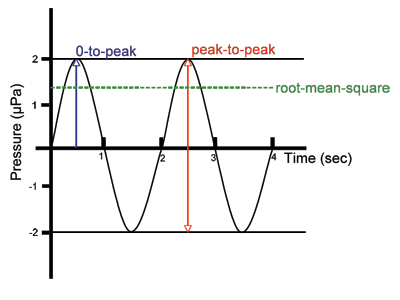# Units Page for Regulators

## Fact Sheet on Units for Regulators

There are fixed units such as meters and relative units such as decibels (dB). Relative units are related to specific conditions. For example, decibels in water have a different relative value than decibels in air. Scientists have agreed to use 1 microPascal (μPa) as the reference pressure for underwater sound. In air, however, scientists have agreed to use a higher reference pressure of 20 microPascals.

Sound intensity given in dB in water is therefore not the same as sound intensity given in dB in air. (Read more)

The standard for measurement is the International System of Units (SI), commonly known as the metric system. SI units are universally used in science and engineering. While the United Sates recognizes SI units as the international standard, it is voluntary to use metric units in the US. Most people in the United States use US customary units, such as the pound, gallon, foot, and Fahrenheit, on a daily basis. However, US customary units are defined in terms of SI units.

Below are some common units used in underwater sound, what they are used to measure, and how they can be converted between each other.

### Units Related to the Measurement of Sound

#### Frequency

1 hertz (Hz) = 1 cycle per second – a unit of frequency
1 kilohertz (kHz) = 1,000 hertz

#### Pressure

1 pascal (Pa) – a unit of pressure
1 micropascal (μPa) = 10-6 Pa – commonly used when measuring sound pressures.
1 atmosphere (atm) = 14.7 lbs/in2 = 101,325 Pa

#### Intensity

decibel (dB) – a measure of the intensity of a sound; 1/10 of a Bel.
Decibels are a relative unit comparing two pressures; therefore, a reference pressure must also be indicated. In underwater acoustics, the reference pressure is 1 micropascal, so the true unit of intensity for underwater sound is dB referenced to 1 micropascal. In air, scientists have agreed to use a higher reference pressure of 20 micropascals. Therefore, the true unit of intensity for sounds in air is dB referenced to 20 micropascal.

Because they use different reference pressures, sound intensity given in dB in water is not the same as sound intensity given in dB in air.

#### Sound Pressure Level (SPL)

SPL is a means of characterizing the amplitude of a sound based on pressure measurements that can be done in several different ways.
-root-mean-square pressure (rms pressure) = the square root of the average of the square of the pressure of the sound signal over a given duration.
-peak-to–peak pressure = the range in pressure between the most negative pressure and the most positive pressure of the signal.
-peak pressure = the range in pressure between zero and the greatest pressure of the signal; also called 0-to-peak pressureFigure 1: Simple Sound Wave.

#### Sound Exposure Level (SEL)

SEL is the decibel level of the time integral (summation) of the squared pressure over the duration of a sound event; it takes into account both received level and duration of exposure; units are dB re 1 μPa2-s
Single strike SEL = the duration of the sound event is a single pulse or signal; this term is often used in pile driving
SELcum = the duration of the sound event is multiple pulses or signals, which should be specified as well as the total duration over which the sound energy is accumulated

### Other Common Units

#### Distance

1 meter (m) – a unit of distance; equal to 3.28 feet
1 kilometer (km) = 1,000 meters
1 centimeter (cm) = 0.01 meters (100 cm = 1 m)
1 millimeter (mm) = 0.001 meters (1000 mm = 1 m)
1 micrometer (μm also 1 micron) = 10-6 meters

1 nautical mile (nm) = 1.85 km or 1.15 miles

US Customary units
1 foot (ft) – a unit of distance; equal to 12 inches
1 inch = 2.54 cm
1 mile = 5,280 feet or 1.6 km

#### Weight/Mass

1 gram (g) – a unit of mass
1 kilogram (kg) = 1,000 grams = 2.2 lbs
1 metric ton = 1,000 kilograms

1 ton – a unit of weight; equal to 2,000 pounds (lbs)

#### Time

1 second (sec) – a unit of time
1 millisecond (msec) = 0.001 seconds (10-3 sec) (1000 msec = 1 sec)
1 microsecond (μsec) = 0.000001 seconds (10-6 sec) (1,000,000 μsec = 1 sec)

#### Temperature

degrees Celsius (°C) and Fahrenheit (°F) – units of temperature

To convert from Celsius (°C) to Fahrenheit (°F):
(°F) = (°C x 1.8) + 32

To convert from Fahrenheit (°F) to Celsius (°C):
(°C) = (°F – 32) ÷ 1.8

#### Salinity

1 part per thousand (ppt) = 1 gram of salt per liter of solution; a unit of proportion equal to 0.001

Practical Salinity Units (PSU or psu) = the conductivity ratio of a seawater sample to a standard potassium chloride solution. Since ratios have no units, it is not true that 35 PSU exactly equals 35 ppt.